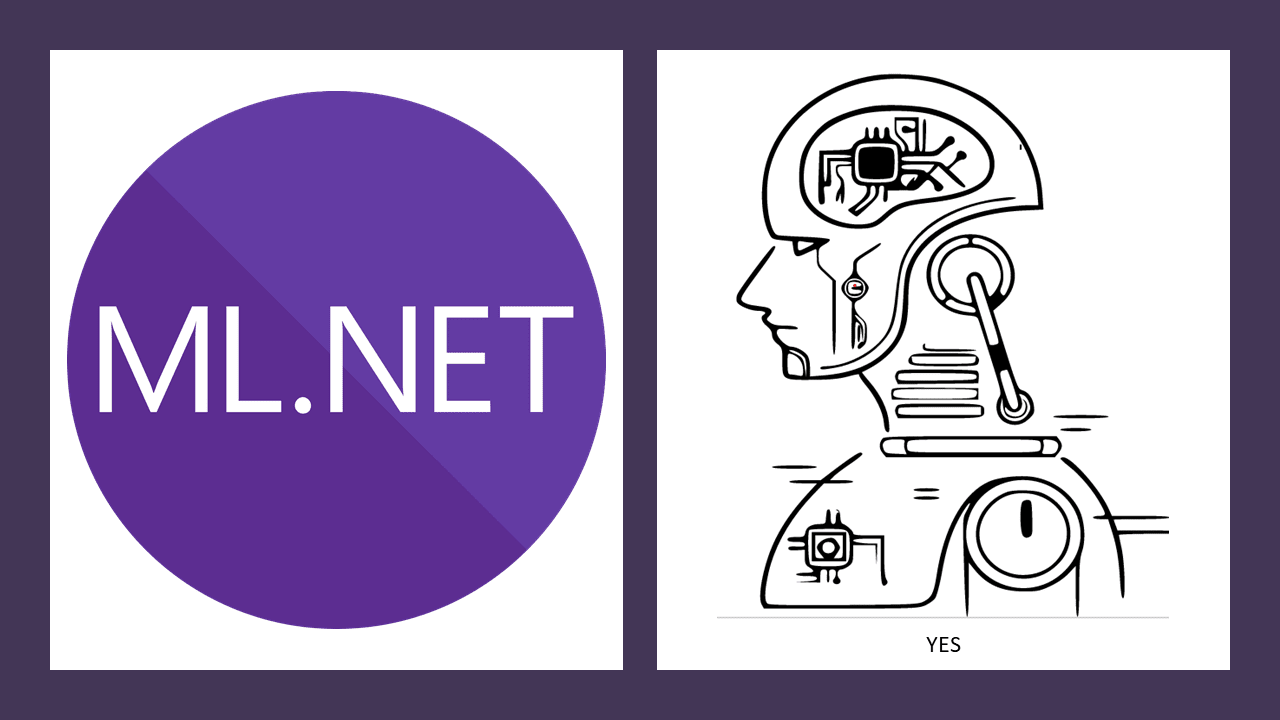# Assumptions of Linear Regression## Linear relationship (Linearity)

This is an expectation that as your independent variables change the dependent variable also changes in a linear fashion.

For example, in a simple linear regression predicting years of experience versus salary the salary reliably increases as years of experience increase. The data has a linear relationship - so in our model we are predicting the rate of increase.

This is typically tested using scatter plots.

## Multivariate normality

This is about the distribution of the data.

So if normal distribution means that the data can be expressed as a bell curve, then multivariate normality means that all of the the independent variables can also be understood as a bell curve.

This is not exactly the same as shoving a bunch of variables with normal distribution together. (Although it's possible to do that using a process called Copulae)

This can be tested by looking at a histogram for each variable and looking for the bell curve.

## Low or no multi-collinearity

This means that the independent variables should not have any sort of hidden dependency on each other.

This can be discovered by examining a scatter plot between variable pairs to see if a linear relationship exists.

It's okay if you discover hidden dependency as the method to remedy is usually to remove the dependent variable from the regression pipeline.

## No auto-correlation in the data

This is confusingly similar to multi-collinearity, but it's the correlation of a variable to itself with a time delay. For example, if you had a collection of temperatures taken daily over a month, that variable is correlated with itself as a temperature taken today has a relationship with the temperature taken yesterday.

Another example would be a collection of stock prices from a single stock. The price's current value has a relationship to it's previous value.

## Homoscedasticity

This means that as the data progresses on a linear chart that the data points remain compact not a broad cone as the dependent variable progresses. This can be illustrated with the following example.

Lets say we have family income as the independent variable and luxury spending as the dependent variable.

When income is low luxury spending is low - this is expected. As income grows so does luxury spending - until we get to high levels of income and then luxury spending is highly variable. Some families spend extravagantly while others are more moderate or may never increase luxury spending at all. The data is not homoscedastic.

This can create a wide cone of results which will negatively impact a regression machine learning model.

If you're like me and find these confusing at first then you'll appreciate these links. These really helped me understand these requirements better.

Assumptions of Linear Regression

What is Multicollinearity

What is Autocorrelation

Multivariate Normal Distribution

Homescedasticity